## Friday, December 14, 2012

### Random Numbers and Polynomials

Random Numbers and Polynomials

Let R be a random number such that 0 ≤ R ≤ 1. Then the inequality by x four times. Then:

0 ≤ R ≤ 1

0 ≤ R x ≤ x

0 ≤ R x^2 ≤ x^2

0 ≤ R x^3 ≤ x^3

0 ≤ R x^4 ≤ x^4

And for any integer n,

0 ≤ R x^n ≤ x^n

If we try to graph R x on a graphing calculator, we can imagine that the calculator generates a random R between 0 and 1 for each x. Graphing four y = R x on top of each other produces a result like this:Here are the graphs for y = R x^2, and y = R x^3, respectively.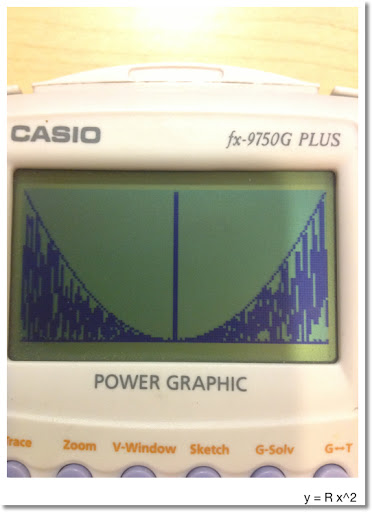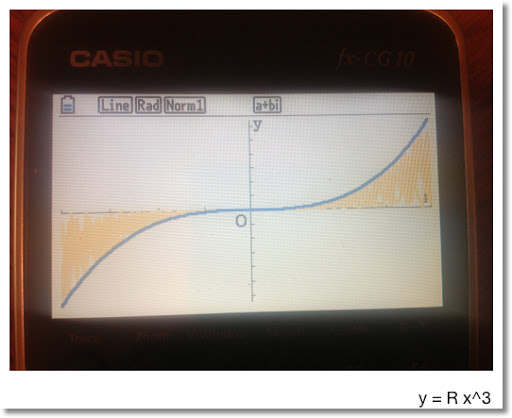Note that:

For odd n and x ≥ 0:

0 ≤ R x^n ≤ x^n

For odd n and x < 0:

0 ≥ R x^n ≥ x^n

For even n:

0 ≤ R x^n ≤ x^n

An Interesting Thing When Building Polynomials

Consider the function: y = Rx^2 + Rx + R

We can look at this two ways:

1. Treat R as a constant random number. Then y = R(x^2 + x + 1)

2. Treat each R as a separate random number, 0 ≤ R ≤ 1. In fact, when you put the function y = Rand# x^2 + Rand# x + Rand#, the calculator treats Rand# as a separate command.

Let's look at a comparison, where y = Rand# (x^2 + x + 1) is in red and y = Rand# x^2 + Rand# x + Rand# is in blue. Recall that Rand# is a random number function.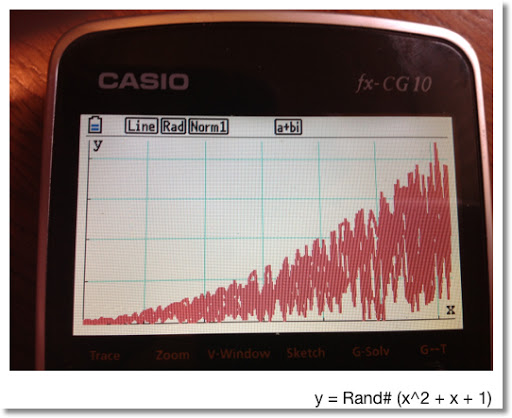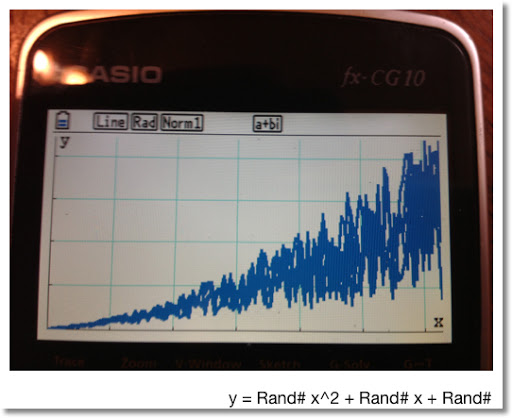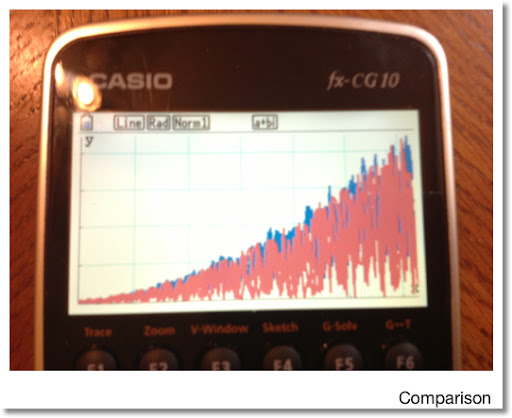That is it. Stay safe everyone, it is a crazy world! Eddie

This blog is property of Edward Shore. 2012